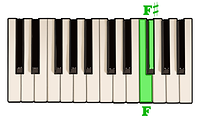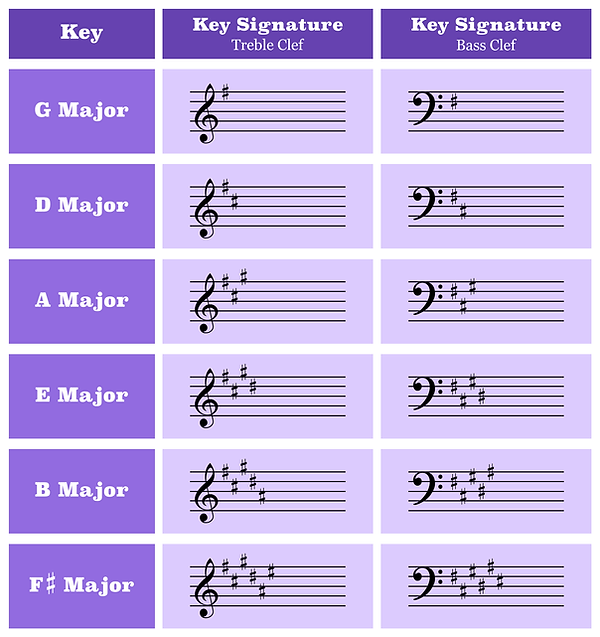top of page

## Understanding Major Scales And Key Signatures

A scale is a group of notes arranged in a definite pattern of whole steps and half steps.

All of the notes in a scale relate back to one common tone called the key note. For instance, the key note of a D Major scale is the note D. This simply means that all of the notes in the D Major scale together as a whole are in the key of D Major.

The arrangement of steps which constructs a Major scale is as follows:Let’s use this information to put together a C Major scale.

Remember from the last video lesson that B and C and E and F are the only two sets of natural notes which are separated by a half step.

Starting on C and following the correct order of whole steps and half steps a C Major scale would look like this:As you can see, the C Major scale is made up entirely of natural notes. It is in fact the only Major scale which does not require the use of a single sharp or flat. Every other Major scale will contain at least one accidental. However, if we stick to the correct pattern of whole steps and half steps they will not be hard to figure out.

The first scale which contains a sharp is the G Major scale. When playing the notes of a G Major scale remember to follow the correct order of whole steps and half steps.*We know that the notes E natural and F natural are separated by one half step. Therefore, when wanting to play a whole step above the note E we must raise the note F natural up one half step to the note F-sharp.A half step above the note E is the note F.A half step above the note F is the note F-sharp.Therefore, a whole step above E is F-sharp.

The key signature is a combination of sharps or flats placed at the beginning of a staff before the time signature which indicates the key of a particular piece of music.

For instance, we know that the only sharp used in the construction of a G Major scale is the note F-sharp.F-sharp, being the only accidental in the G Major scale, can therefore be considered the key signature for the key of G Major.When we put the F-sharp in the beginning of the staff after the clef this implies that every note which is normally read as F-natural is actually the note F-sharp, regardless of whether or not there is a sharp directly next to the note or not.

To find the key signature of any particular key combine only the notes from the scale which contain an accidental.

Here is a list of each key signature which contains only sharps.In order to understand the key in which each key signature is representing take the last accidental in the key signature and go up one half step. For instance, one half step above C-sharp is D. F-sharp, C-sharp is the therefore the key signature to D Major. Likewise, one half step above A-sharp is B. Therefore, F-sharp, C-sharp, G-sharp, D-sharp, A-sharp is the key signature of B Major.

Here now is a list of each key signature which contains only flats.With the order of flats, the key in which the key signature is representing is always the second to last accidental. The second to last accidental is B-flat, E-flat, A-flat is E-flat. Therefore B-flat, E-flat, A-flat is the key signature of E-flat. The only exception to this rule is the key of F Major. The key signature of F Major, B-flat, does not follow these guidelines.

bottom of page